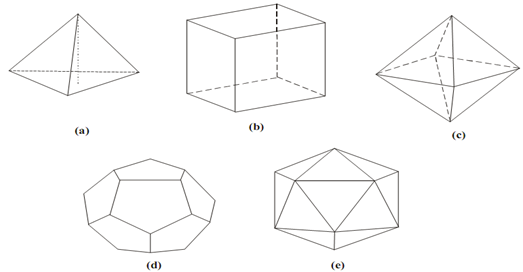## Different Types of Solid Assignment Help

Assignment Help: >> Orthographic Projections - Different Types of Solid

Different Types of Solid:

A solid is a three-dimensional object containing length, thickness and breadth. It is completely bounded through surfaces such may be curved or plane and accordingly solids may be classified as polyhedra and solids of revolution.

Polyhedra

Polyhedra is defined like a solid bounded through plane surfaces called faces. There are two kinds of polyhedra that are as:

(a) Regular, and

(b) Oblique or Solid

Regular Solid or Regular Polyhedra

A regular polyhedron is a solid bounded through faces that are equal and usual, as displayed in diagram.Tetrahedron

It has four equivalent faces, each an equilateral triangle in diagram (a).

Cube or Hexahedron

It has six equivalent faces, each a square as in diagram (b).

Octahedron

It has eight equivalent faces, each an equilateral triangle in diagram (c).

Dodecahedron

It has twelve equivalent faces, each a regular pentagon as in diagram (d).

Isosahedron

It has twenty equivalent faces, each an equilateral triangle in diagram (e).

 Irregular or Oblique Polyhedra Prism Pyramid